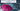# First Unique Character in a String - Leet Code Solution

September 08, 2020## Problem Statement

Given a string, find the first non-repeating character in it and return its index. If it doesn’t exist, return -1.

Example

``````s = "leetcode"
return 0.

s = "loveleetcode"
return 2.``````

Note: You may assume the string contains only lowercase English letters.

## Solution Brute Force

Lets take a look at the simple solution.

• Have two loops. first will iterate till length of string
• in second loop, iterate from beginning to end.
• And check if the character is found anywhere
• We can keep track of duplicate found by a boolean flag
• And if during our inner loop, if we found that character is not found. This is our answer

### Code

``````public int firstUniqChar_bruteforce(String s) {
for (int i=0; i<s.length(); i++) {
boolean unique = true;
for (int j=0; j<s.length(); j++) {
if (i != j && s.charAt(i) == s.charAt(j)) {
unique = false;
break;
}
}

if (unique) {
return i;
}
}

return -1;
}``````

### Complexity

Its `O(n^2)`

## Another Solution using a HashMap

• Iterate over string, and keep track of count of each character
• Maintain a `HashMap<Character, Integer>`
• Now, iterate over string again
• For each character, lookup in our `HashMap`
• If the count of that character is only `1`, this is our answer
• Since, `1` means this character is in the string only 1 times.

### Code

``````public int firstUniqChar(String s) {
Map<Character, Integer> map = new HashMap<Character, Integer>();
for (int i=0; i<s.length(); i++) {
int count = map.getOrDefault(s.charAt(i), 0);
count ++;
map.put(s.charAt(i), count);
}

for (int i=0; i<s.length(); i++) {
if (map.get(s.charAt(i)) == 1) {
return i;
}
}
return -1;
}``````

### Complexity

Its `O(n)`